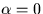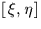Next: SLA_TPS2C - Plate centre from and
Up: SUBPROGRAM SPECIFICATIONS
Previous: SLA_TP2S - Tangent Plane to Spherical

## SLA_TP2V - Tangent Plane to Direction Cosines

ACTION:
Given the tangent-plane coordinates of a star and the direction cosines of the tangent point, determine the direction cosines of the star (single precision).

CALL:
CALL sla_TP2V (XI, ETA, V0, V)

GIVEN:

 R XI,ETA tangent plane coordinates of star (radians) V0 R(3) direction cosines of tangent point

RETURNED:

 R(3) V direction cosines of star

NOTES:
1.
If vector V0 is not of unit length, the returned vector V will be wrong.
2.
If vector V0 points at a pole, the returned vector V will be based on the arbitrary assumption thatat the tangent point.
3.
The projection is called the gnomonic projection; the Cartesian coordinatesare called standard coordinates. The latter are in units of the distance from the tangent plane to the projection point, i.e. radians near the origin.
4.
This routine is the Cartesian equivalent of the routine sla_TP2S.Next: SLA_TPS2C - Plate centre from and
Up: SUBPROGRAM SPECIFICATIONS
Previous: SLA_TP2S - Tangent Plane to Spherical

SLALIB --- Positional Astronomy Library